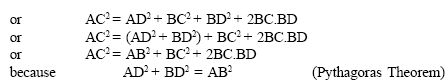Email us to get an instant 20% discount on highly effective K-12 Math & English kwizNET Programs!

#### Online Quiz (WorksheetABCD)

Questions Per Quiz = 2 4 6 8 10

### Geometry2.25 Pythagorean Theorem - Examples

 Theorem: In a right angled triangle, the square on the hypotenuse is equal to the sum of the squares of the other two sides. If the length of the hypotnuse is c and the lengths of other two sides are b and a then according to the pythogorean theorem: c2 = a2 + b2Converse: In a triangle if the square of one side is equal to the squares on the sums of the other two sides, then the angle opposite to the first side is a right angle. To prove: AB2 + BC2 = AC2Example: Prove that the sum of the squares of the sides of a rhombus is equal to the sum of the squares of the diagonals.Directions: Solve the following problems. Also write at least 10 examples of your own.
 Q 1: Triangle PQR is equilateral. QR = 30 units, B is the midpoint of QA. QA is perpendicular to PR. What is the length of PB? 23 cm19.84 cm24 cm Q 2: A ladder 20m long reaches height of 16m above the ground. Find the distance of the foot of the ladder from the wall.14 m12 m13 m Q 3: A ladder 20 m long reaches a height of 16m above the ground. Find the distance from the foot of the ladder to the wall.17 m12 m13 m Q 4: A ladder is placed in such a way such that its distance from the wall to the foot of the ladder is 5 cm, its top reaches a height of 12m from the ground. Find the length of the ladder.15m12m13m Q 5: The sides of a triangle are 7cm, 24cm, 25cm, check if this is a right angled triangle.yesno Q 6: A person goes 10 m due east and then 30m due north. Find his distance from the starting point.10m12m15m Q 7: Two poles 6m and 11m stand on a plane ground. If the distance between their feet is 12m, determine the distance between their tops.12 m13 m11 m Q 8: The sides of a triangle are 50cm, 80cm, 100cm, check if this is a right angled triangle.yesno Question 9: This question is available to subscribers only! Question 10: This question is available to subscribers only!